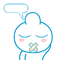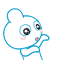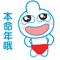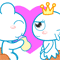# MySQL 中的联合索引介绍【最左前缀匹配原则】

【摘要】在进行多条件查询时，我们不可避免的会用到联合索引，在实际开发的使用是非常频繁的。mysql 的联合索引有称为复合索引、组合索引，而讲到联合索引一定是离不开最左前缀匹配原则的，本文通过举些简单的例子，来讲解联合索引中的最左匹配原则。联合索引优化的目标就是覆盖索引，即在使用 sql 查询的时候要尽快能的使所有的字段都能够被利用。

1. `create table union_index(`
2. `	id int auto_increment primary key,`
3. `	a int,`
4. `	b int,`
5. `	c int,`
6. `	d int,`
7. `	index abc(a,b,c)`
8. `);`

## 一、多列索引在 and 查询中的使用

1. `-- 查询效率最高,索引全部覆盖（口诀：全值匹配我最爱）`
2. `select * from union_index where a = 1 and b = 1 and c = 1; `
3. `-- 覆盖索引 a 和 b`
4. `select * from union_index where a = 1 and b = 1; `
5. `-- 经过 mysql 查询分析器的优化，覆盖索引 a 和 b`
6. `select * from union_index where b = 1 and a = 1; `
7. `-- 只覆盖最左的索引字段 a`
8. `select * from union_index where a = 1; `
9. `-- 没有走索引,最左字段 a 不存在（口诀：带头大哥不能死）`
10. `select * from union_index where b = 1; `
11. `select * from union_index where b = 1 and c = 1; -- 同上`
12. `select * from union_index where c = 1; -- 同上`
13. `-- 覆盖索引 a （c 没有覆盖，因为中间的 b 没使用到）（口诀：中间兄弟不能断）`
14. `select * from union_index where a = 1 and c = 1; `
15. `-- 覆盖索引 a,非联合索引字段 d 不涉及`
16. `select * from union_index where d = 1 and a = 1; `

## 二、多列索引在范围查询中的使用

1. `-- （口诀：范围之后全失效）`
2. `-- 只覆盖索引 a 和 b,但 b 列是范围查询,所以字段 c 不走索引`
3. `select * from union_index where a = 1 and (b between 1 and 10) and c = 1; `
4. `-- 覆盖索引 a ,a 是范围查找,因此 b 和 c 无法使用索引`
5. `select * from union_index where a > 1 and b = 1 and c = 1; `
6. `-- b 是范围查询,但 b,c 都不走索引，因为最左字段 a 没用到`
7. `select * from union_index where b > 1 and c = 1; `
8. `-- 经过 mysql 查询分析器的优化：a = 1 and b = 1 and c > 1,因此覆盖索引 a 和 b 和 c`
9. `explain select * from union_index where a = 1 and c > 1 and b = 1; `
10. `-- 覆盖索引 a,d 是非联合索引字段,无关紧要`
11. `select * from union_index where d > 1 and a = 1; `

1. `-- order by 排序中用到联合索引最左字段 a,但没有 where 条件做铺垫,故不走任何索引,会出现 Using filesort`
2. `select * from union_index order by a asc; `
3. `-- 覆盖索引 a`
4. `select * from union_index where a = 1 order by a asc; `
5. `-- 覆盖索引 a 和 b,想象成字段 b 是继承了 where 条件中 a 后面`
6. `select * from union_index where a = 1 order by b asc; `
7. `-- 只使用索引 a,字段 b 中断了，故无法使用索引 c,(同样遵守最左优先)，会出现 Using filesort`
8. `select * from union_index where a = 1 order by c asc; `
9. `-- 覆盖索引 a 和 b 和 c,不会出现 Using filesort`
10. `select * from union_index where a = 1 and b = 1 order by c asc; `
11. `-- 覆盖索引 a 和 b 和 c,不会出现 Using filesort`
12. `select * from union_index where a = 1 order by b,c asc; `
13. `-- 不走任何索引,会出现 Using filesort`
14. `select * from union_index where b = 1 order by c asc; `
15. `-- 覆盖索引 a 和 b,b 和 c 的排序顺序不同,所以不走索引 c，会出现 Using filesort`
16. `select * from union_index where a = 1 order by b desc,c asc; `

1. `-- 这种情况在 order by 中是不走索引的，但是在 group by 中是利用索引了`
2. `select * from union_index group by a; `

LIKE百分写最右,覆盖索引不写星(*)；

0

0

 评论审核未开启 回复后邮件通知我• 浏览最多
• 评论最多